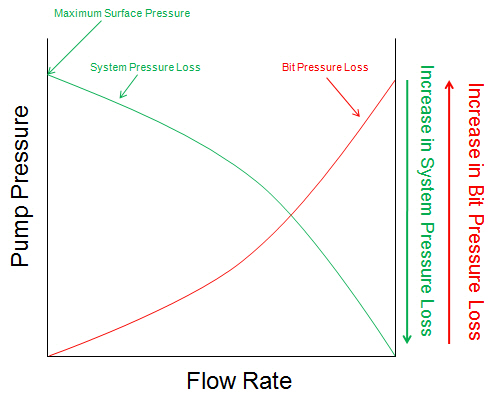## Determine The Optimized Points for Drilling Hydraulics

The design of hydraulic optimization begins with downhole component and an analysis of pressure losses as a function of flow rate.

Before I will explain you about the pressure and flow rate curve, I would like to get the following term across. The surface pressure (pump pressure) is equal to the system pressure loss and the pressure loss across the bit.

P surface = P system + P bit

The curve below demonstrates a system pressure loss curve and a pressure loss across the bit as Y-axis and a flow rate as X-axis. The system pressure loss curve starts at the maximum surface pressure and is drawn invert with the bit pressure loss curve (see the curve below more understanding).